In this lesson, we will learn:

• How to add decimal numbers using base ten (block) models
• That adding decimal numbers is the same as adding whole numbers, which involves lining up the right place values and regrouping

Notes:

• To add decimals with base ten (block) models:
• Remember the three types of blocks used to represent the ones, tenths, and hundredths place values
• "One whole" or hundred block = ones place value
• Column (stick or rod) = tenths place value
• Single square = hundredths place value• Count all of the blocks that you are adding up, altogether
• Regroup to get to your final answer. 10 of any type of block will regroup into the next bigger type of block.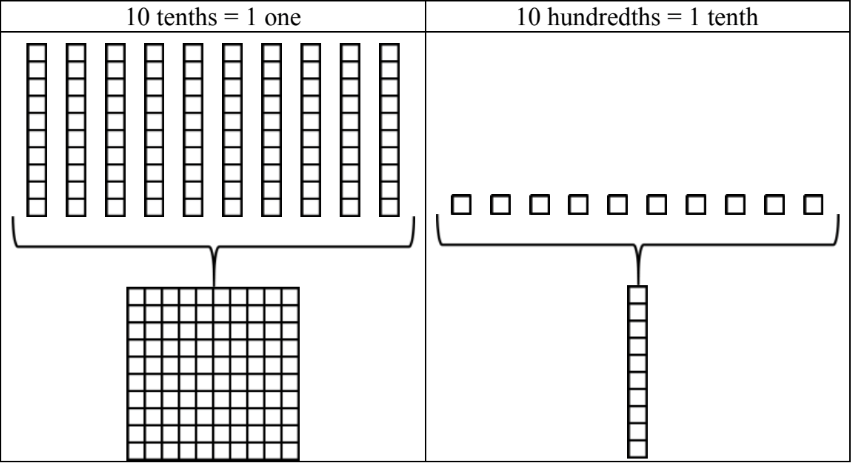• To add decimals, line up the decimal point to make sure you are adding the right place values in the same columns. Regroup (carry over) numbers whenever necessary.
• Sometimes, you will have to write one or more zeroes to a number after the decimal point (trailing zeroes) when the addends don't have the same number of place values.

#### Lessons

• Introduction
a)
How to add decimals with base ten (block) models

• 1.
Regrouping practice for decimals with block models
Regroup so that each place value only has one digit. Use base ten (block) models to help.
a)
17 tenths = ____ ones + ____ tenths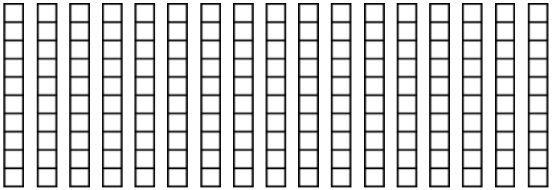b)
4 ones + 12 tenths + 39 hundredths = ____ ones + ____ tenths + ____ hundredths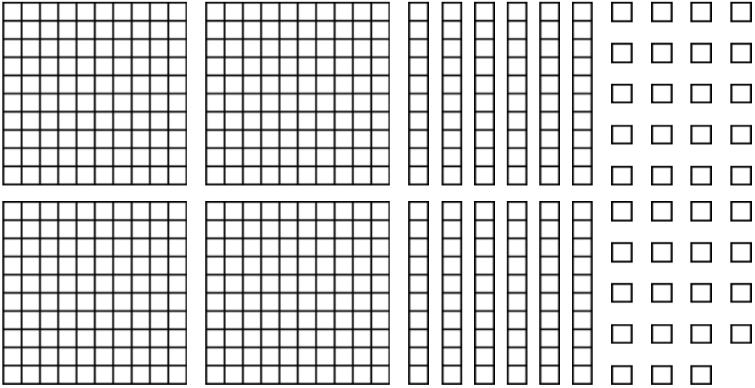c)
6 ones + 29 tenths + 16 hundredths = ____ ones + ____ tenths + ____ hundredths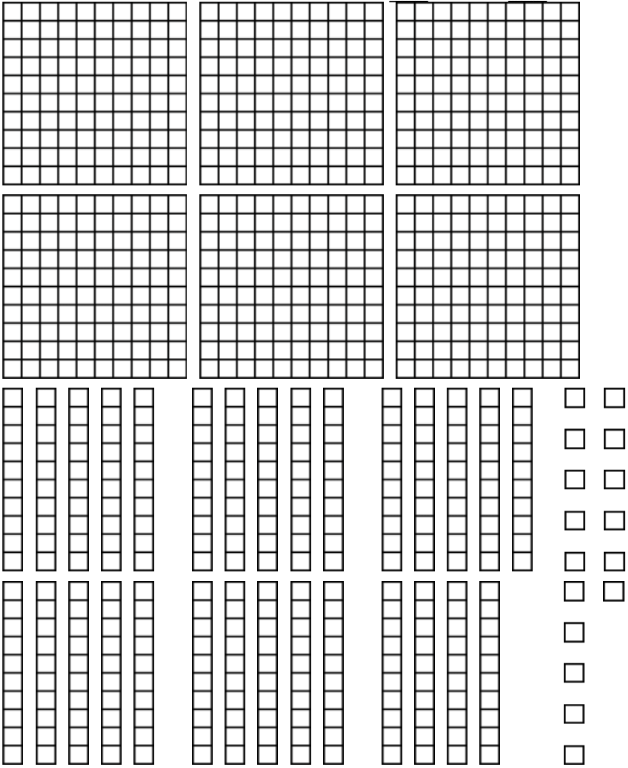• 2.
a)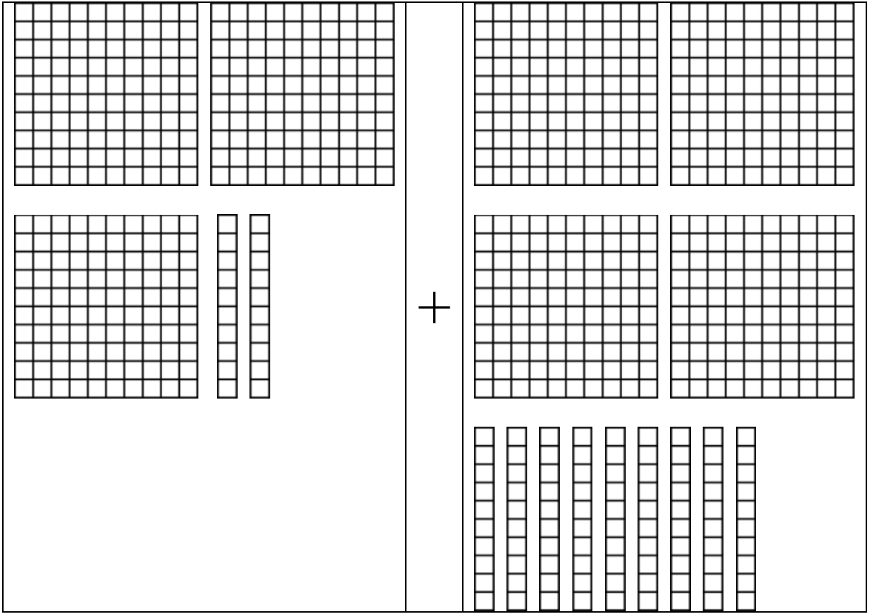b)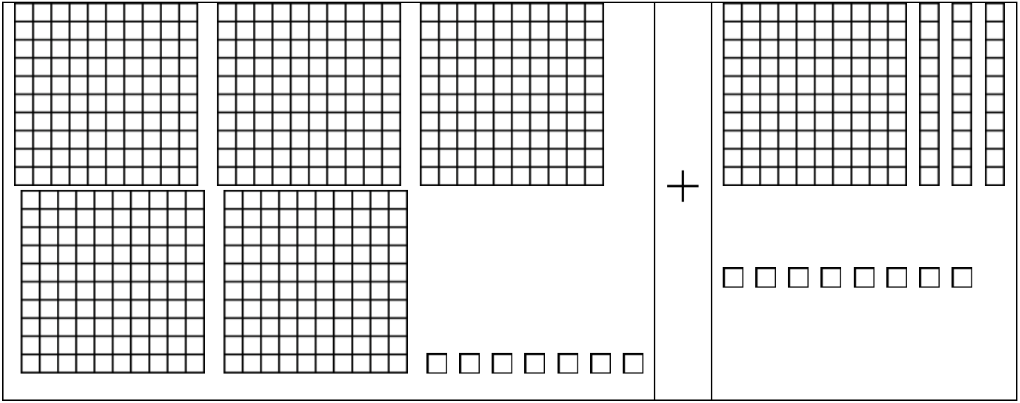c)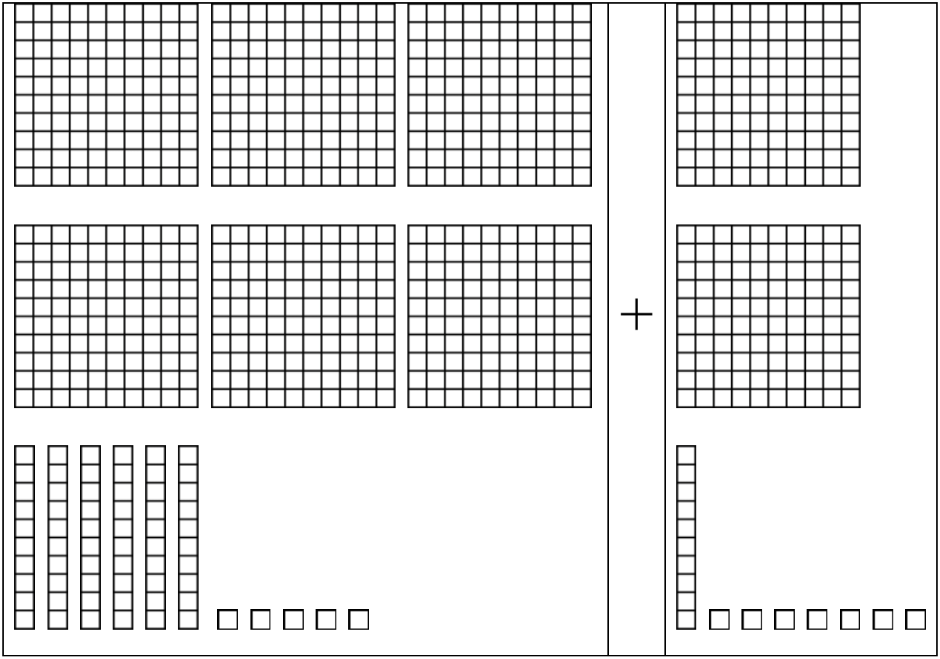• 3.
Add the decimals by lining up on the grid. Regroup where necessary.
a)
34.8 + 5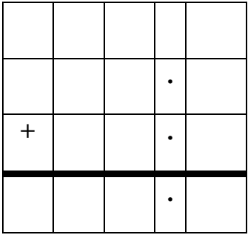b)
4.6 + 2.9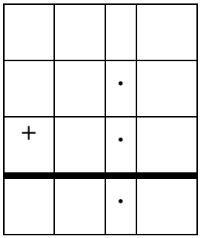c)
41.03 + 5.72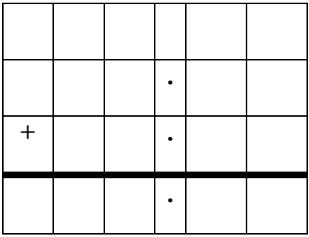d)
35.84 + 6.5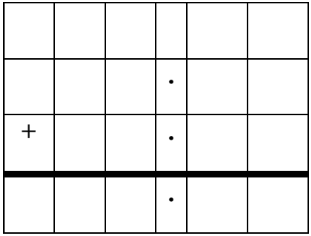• 4.
Adding decimals and rounding decimal sums
a)
7.6 + 2.14

b)
80.65 + 19.23

c)
18.24 + 3.17

d)
98 + 38.97